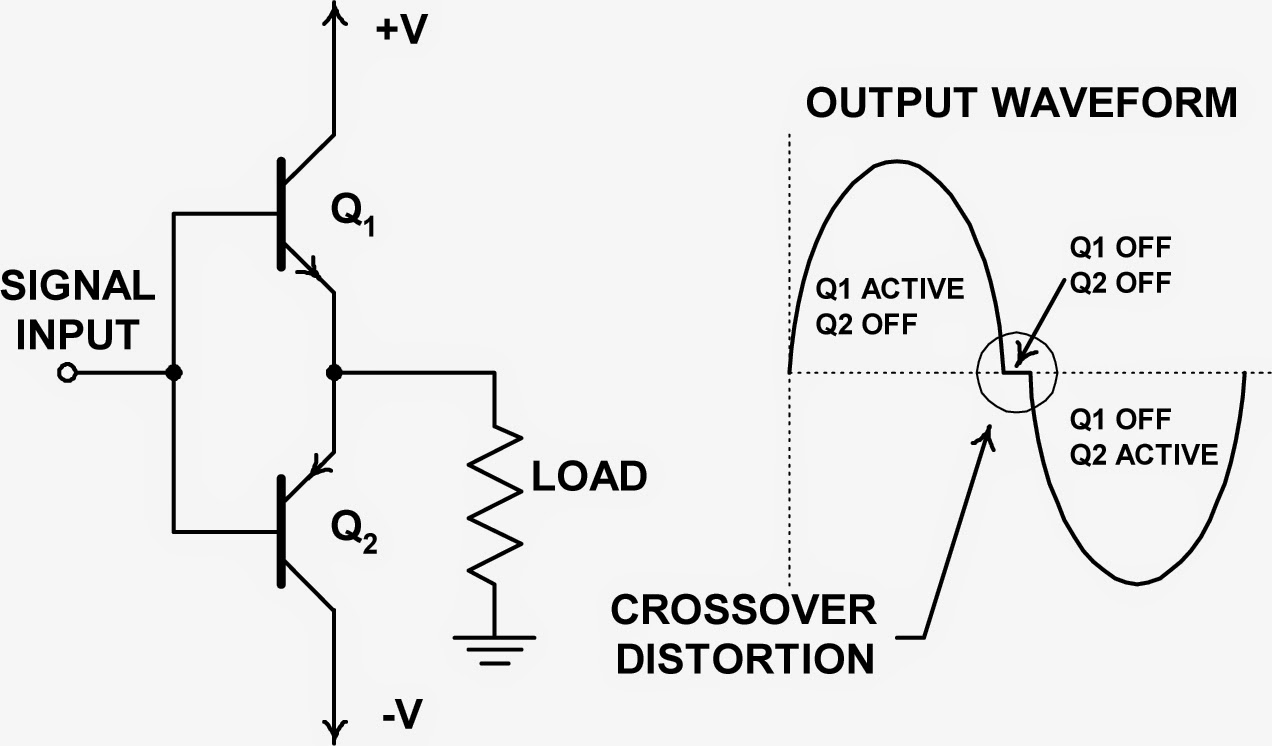## You need to test, we're here to help.

You need to test, we're here to help.

## 20 March 2015

### Using Histograms (Part III)Figure 1: A simplified view of a push-pull amplifier showing the source of crossover distortion
In this third post in a series on using an oscilloscope's histogram capabilities, let's take a look at using histograms as a diagnostic tool. Diagnosing problems in a circuit calls for good skills and some intuition on top of good measurement tool. In general, though, the more ways in which you're able to look at a problem, the more likely it is that you'll turn up the root cause.

Many oscilloscopes, including Teledyne LeCroy's, offer frequency and statistical domain processing that can be of great utility to troubleshooters. These capabilities provide alternate views of the acquired data that in turn present addition perspective for diagnosis.Figure 2: A time-domain waveform with crossover distortion
Consider the example of detecting and diagnosing crossover distortion in a push-pull amplifier stage (Figure 1). Crossover distortion—particularly at low levels—is tough to see in a conventional oscilloscope time-domain display of the waveform. Figure 2 shows an example of a waveform plagued with crossover distortion. Even though the distortion is significant, it is barely visible in the screen capture and could easily be overlooked.Figure 3: The FFT shows high harmonic content due to distortion but does not help determine its root cause
Many modern oscilloscopes provide additional signal-processing functions such as fast-Fourier transforms (FFTs) with which to discern signal problems such as distortion. The FFT is a great tool in determining whether a signal harbors distortion as shown in Figure 3. But an FFT cannot, however, differentiate between sources of distortion. Clipping, asymmetries, limiting, and crossover distortion all produce similar frequency-domain spectra. The high harmonic content, which in this case is about -45 dB down relative to the fundamental, is a key indication of the presence of distortion in the waveform.Figure 4: The histogram of data amplitude (lower left) reveals a higher-than-normal number of sample values at the zero crossing.
However, the histogram of the amplitude data values, which are added to the waveform and FFT in Figure 4, tells a more revealing story about the nature of the distortion present in our waveform.

Histograms are created by dividing the amplitude range of the oscilloscope into 20 to 5000 bins (100 bins are used in this example). The number of samples falling into each bin is plotted, on the vertical axis, against the nominal voltage value of the bin on the horizontal axis. The histogram of the data values shows the number of samples in the waveform within each small voltage range.

In Figure 4, the histogram in blue at lower left is of our signal with crossover distortion while the plot in yellow at lower right is of a properly functioning amplifier. Now we are able to compare them. As seen in the yellow histogram of a sine wave, the histogram assumes a shape not unlike that of a saddle. But the blue histogram shows us an abnormal number of sample values at the center, which represents the zero-volt crossover. The waveform is hesitating at the zero crossing, which is a sure sign of crossover distortion. If our signal was clipping or limiting, we would see this manifested in the histogram as a higher-than-normal population at the maximum and/or minimum peaks. Distortion caused by asymmetry would appear as an asymmetric histogram plot.

Thus, we see that the ability to view a signal in the time, frequency, and statistical domains is a very powerful tool in the diagnosis of complex circuit aberrations.